# ZHOU AND TUNG

In a recent posting I said I would be commenting on a paper by Zhou and Tung (Zhou, J., and K. Tung, 2012: Deducing Multi-decadal Anthropogenic Global Warming Trends Using Multiple Regression Analysis. J. Atmos. Sci.doi:10.1175/JAS-D-12-0208.1, in press.)

When I came across this paper I had mixed feelings. The paper says very similar things to those have I have been saying since January 2012: that the underlying rate of temperature increase is less than IPCC models assume, due to the influence of the Atlantic Multidecadal Oscillation (AMO). I was pleased to get further corroboration in a peer reviewed paper. On the other hand I was peeved as a paper I submitted earlier this year was not accepted.

Their approach is similar to that of Foster and Rahmstorf.

Forster and Rahmstorf developed a multiple linear regression model using total solar radiation, aerosols, ENSO and a linear trend as independent variables and 5 alternate temperature records as the dependent variable. The period analysed was 1979 to 2010; the only period common to all 5 temperature series. They concluded that for that the period the underlying temperature trend was 0.014 to 0.18 °C per year. The paper was welcomed in many quarters as countering the claim that the rate of temperature increase had had been falling off or even stationary for the last decade or more of that period.

The Zhou and Tung paper adopts a similar approach but they have substituted the ENSO with the AMO. They conclude that the rate of temperature increase since the start of the 20th century, which they ascribe to anthropogenic effects, has been less that that estimated by Foster and Rahmstorf. They give 0.0068 °C for the 100 year trend, 0.0080 °C for the 75 year trend, 0.0083 °C for the 50 year trend and 0.0070 °C for the 25 year trend. These figures are about half of those of Foster and Rahmstorf.

They consider the suggestion of Booth et al that the AMO is anthropogenic and reject it.

My own equivalent figures are 0.0050 °C per year from 1856 to the present, 0.0067 °C for the 100 year rate and 0.011 for the 30 year rate. These values are similar to those of Zhou and Tung with one exception: I get an accelerating rate of increase which reflects the growing concentration of GHGs.

The conclusion of both their work and mine is the same: climate models, which simulate all the increase in temperature as anthropogenic and driven by GHGs, are overestimating the increase in temperature by a factor of two. A corollary to both sets of ideas is that if, as seems likely, the AMO is regular then it is likely to restrict temperature increase for the next few decades while the AMO is decreasing.

# AMO AND ANTHROPOGENIC AEROSOLS

It has been pointed out that the model I described in my earlier post (Climate and the Atlantic Multidecadal Oscillation) ignored anthropogenic aerosols. Here I look at the effect of adding these into the model.

Data
The data used were downloaded from http://data.giss.nasa.gov/modelforce/Fe.1880-2011.txt. They were used in J. Hansen, et al. (2007) "Climate simulations for 1880-2003 with GISS model E", Clim Dyn, 29: 661-669 and J. Hansen, et al. (2011) "Earth's energy imbalance and implications, Atmos Chem Phys, 11, 13421-13449.

Figure 1 shows the individual components.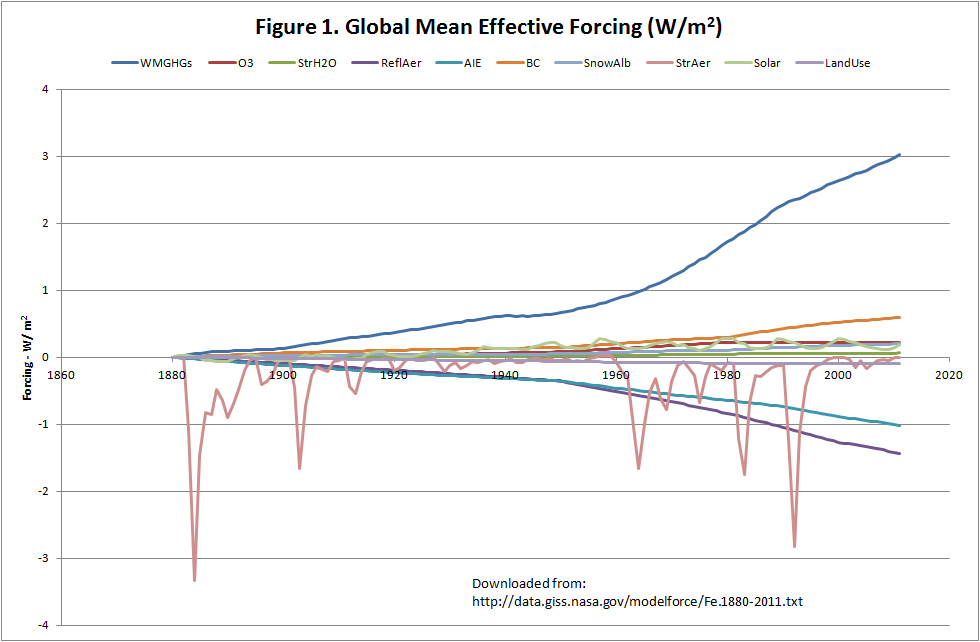The individual components are:

WMGHGs – Well mixed greenhouse gases
O3 - Ozone
StrH2O – Stratospheric H­2O
ReflAer – Reflective Aerosols
AIE – Aerosol indirect effect
BC – Black carbon
SnowAlb – Snow albedo
StrAer – Stratospheric Aerosols (Volcanoes)
LandUse – Land Use.

To run a regression model with these 10 parameters plus the Atlantic Multidecadal Oscillation (AMO) would be nonsense. This would be particularly so since many of the components are highly correlated; the regression coefficient between WMGHGs and AIE is 0.98 for example. So I aggregated them into 4 groups. The first three (WMGHGs, O3 and StrH2O) I grouped as GHGs. Stratospheric aerosols and solar were treated separately. This gave 3 parameters which had exact equivalent in the original 4-parameter model. The fourth parameter was the sum of all the other components. The aggregated parameters are shown on Figure 2.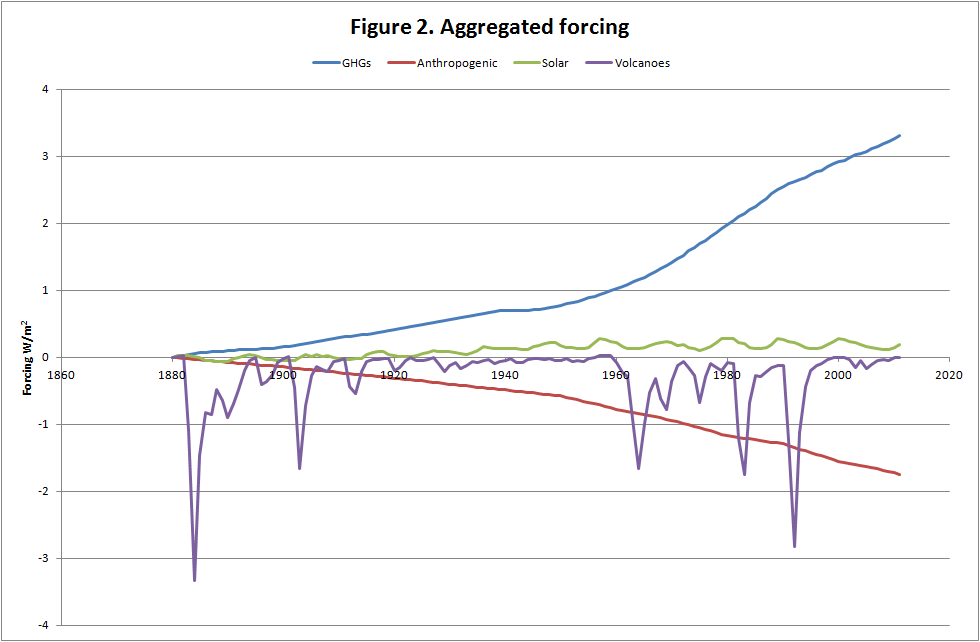The fifth and final parameter was the AMO.

For temperature I used the HadCRUT3 global data set. I am aware that this has been superseded by the version 4 but for consistency with the earlier posting I am sticking to it.

Results
The accuracy of the two models was almost identical. This can be seen on figure 3.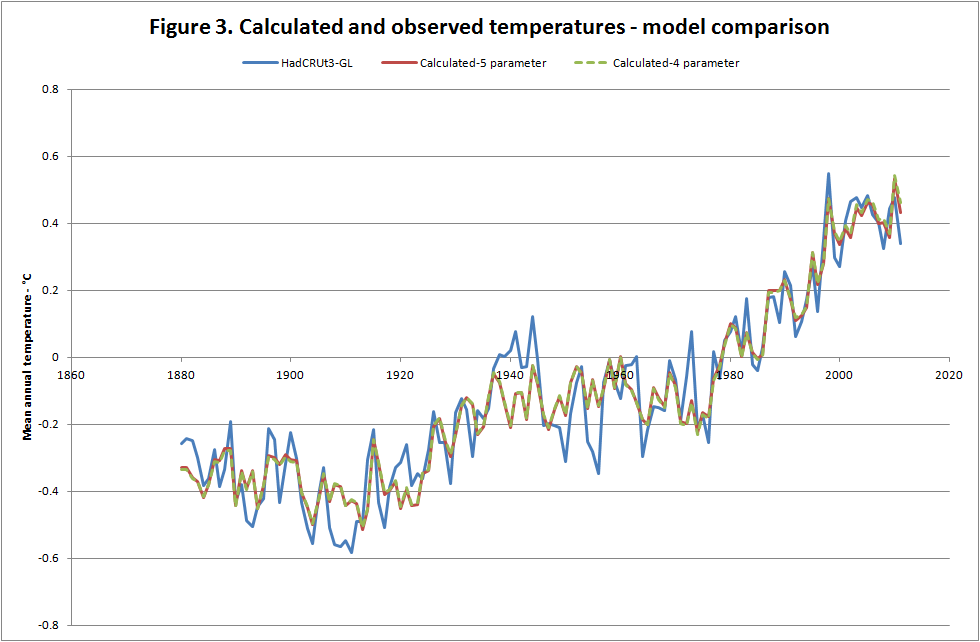In terms of accuracy, the 4-parameter model (for the period 1880 to 2011) explained 89.3% of the variance and the 5-parameter model explained 89.5%, confirming the similar accuracy of the two models.

Components
The comparison of observed and calculated temperatures does not describe how the values were actually calculated. Figure 4 shows the effect of each component on temperature.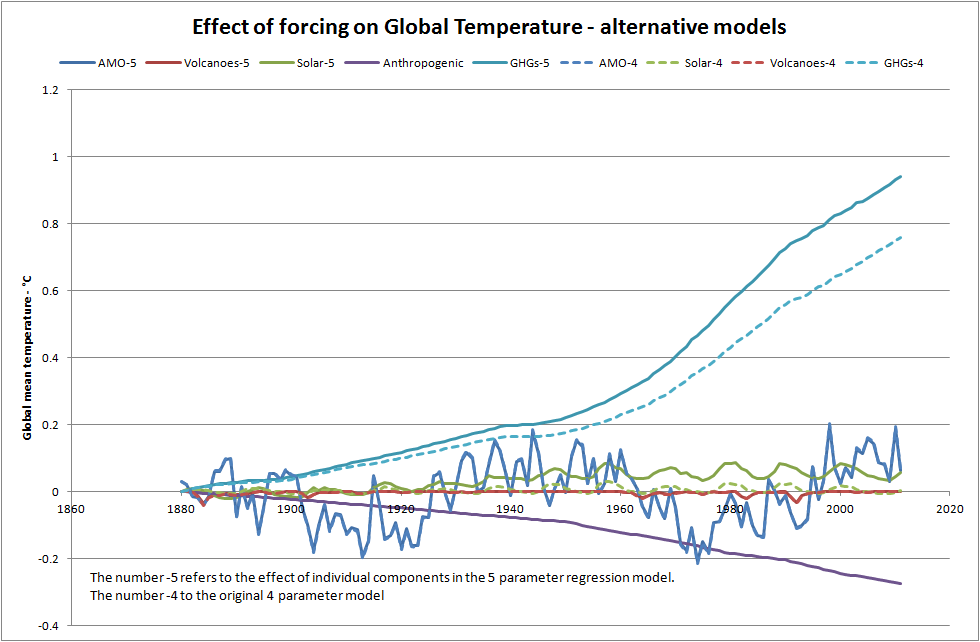In the above the use of ‘-5’ refers to 5 parameter model and ‘-4’ to the original 4 parameter model. The main differences between the models are:

• The effect of GHGs is larger in the 5-parameter model, the difference being largely due to the effect of the anthropogenic factors.
• Solar effects are slightly higher in the 5-parameter models.
• The effect of volcanoes is minimal in both models.
• The influence of the AMO is virtually identical in both models.

The coefficients for the 5 independent variables were:

Since the all parameters, except for AMO, are expressed as W/m2 and if the forcing sensitivity is close to the accepted value of 3.2 W/m2/°C then each coefficient should have the value 0.3125 °C/W/m2 (that is 1/3.2). In this case the parameters for GHGs and solar irradiance are close to that value. That for volcanoes is much lower than expected. The parameter for combined anthropogenic effects is also lower than expected but has wide error bands so the ‘expected’ figure is within the 95% range.

Considering the critical period from 1976 to 2005, which had the largest increase, the observed temperature increases were as given in the table below.

Considering the period of rapid temperature rise, the original 4-parameter model suggested that 49% of the rise was due to GHGs, the equivalent figure for the 5-parameter model is 59%.

These findings are consistent with those reported in Zhou, J., and K. Tung, 2012: Deducing Multi-decadal Anthropogenic Global Warming Trends Using Multiple Regression Analysis. J. Atmos. Sci. doi:10.1175/JAS-D-12-0208.1, in press. I will discuss this paper in another posting.

Conclusions
The conclusion remains as before. It is not clear whether the AMO is the ‘heart’ of the system and is driving global temperature or whether it is the ‘pulse’ and is an indicator another driver. What is clear that global temperatures reflect changes in the AMO but the AOGCMs used by the IPCC do not consider the AMO. In particular the AOGCMs simulated reasonably accurately the large temperature increase from 1976 to 2005 but attribute all the increase to GHGs. As a consequence, as pointed out in this and previous postings, and some recent peer-reviewed papers, temperature increases in the coming decades are likely to be lower than the IPCC projections.

# CLIMATE AND THE ATLANTIC MULTIDECADAL OSCILLATION

1. Introduction

I would guess that whoever you are, if you were to be told that all the 0.75 °C increase in global temperatures over the past 150 years was due to the increase in anthropogenic greenhouse gases but that temperatures over the next three decades would increase by only 0.1 °C you would have mixed reactions. If you were a sceptic you would scoff at the suggestion that greenhouse gases could have such an impact but welcome the suggestion that temperature would only increase marginally. On the other hand if you had built a career, as a climate scientist or a politician, preparing people for large temperature increases you would be horrified at the idea of small temperature increases but be more sanguine about the attribution of the temperature increase to greenhouse gases.

What I describe is a model which seems to suggest that this is in fact the case. Whilst I can’t really believe it myself, I can’t find anything wrong with it. Maybe you can.

This post is about the Atlantic Multidecadal Oscillation (AMO) and its impact on climate.

The AMO is a climatic oscillation with a periodicity of around 60 to 70 years based on the difference between sea temperatures in the North Atlantic and detrended sea temperatures. There are several slightly different definitions. A typical one is based on the difference between the sea temperature in the part of the Atlantic Ocean from the equator to 70°N and the global sea temperature.

Figure 1.1 shows the AMO from 1856 to 2011 based on the work of Enfield et al (2011), downloaded from http://www.esrl.noaa.gov/psd/data/correlation/amon.us.long.data. This version is based on Kalplan sea surface temperatures.There are other oscillation with different periodicities and definitions. Probably the best known of these is ENSO, El Niño/Southern Oscillation. Others include the Pacific Decadal Oscillation and the North Atlantic Oscillation. Whilst these posts will deal primarily with the AMO I will also be considering other oscillations.

The reason that climatic oscillations have attracted attention is because of teleconnection. This refers to the fact that climate changes can be observed in parts of the world remote from the locus of an oscillation but which appear to be related to it. This effect was first identified by Walker (1923) in relation to the Southern Oscillation but similar teleconnections are reported for the other oscillations.

At this point it might be appropriate to introduce a small caveat. Although the timing of a variation in climate might appear to be linked to an oscillation it does not follow that the oscillation caused the climate variation. It is perfectly possible that another phenomenon caused both the variation and the oscillation. The word oscillation implies a regular pattern or variation and although I use the word ‘oscillation’ throughout this article I recognise that the term pseudo-oscillation might be more appropriate. Some of the oscillations which occur over a short time period, a few years in the case of ENSO, are clearly not regular. For the AMO the length of the data is not long enough to establish how regular it is. A similar caveat applies to the use of the word ‘periodicity’.

Many of the sections in this article will be looking at a simple model of global temperature in which the AMO is a parameter. Figure 1.2 shows the simulated and observed global temperature.I will be looking at how this model was developed and some of the alternatives I considered. I will also be looking at the connection between the AMO and hurricanes and sea level.

2. Why are temperatures tending to increase?

The graph of temperature in the preceding post (figure 1.2) shows that they have tended to increase since the start of the global temperature record. In this post I am using the HadCRU3T global data, downloaded from http://www.cru.uea.ac.uk/cru/data/temperature/. This is the longest of the main global temperature indices and takes account of both sea and land temperatures.

The question of why temperatures are increasing is one of the key questions related to climate change. In particular, is the increase due to human influence or is it natural?

Here I consider three possibilities:

Greenhouse gas forcing. The 1995 IPCC report contained the sentence “The balance of evidence suggests a discernible human influence on global climate.” So, this is obviously one of the main candidates. In this case I use Greenhouse Gas Forcing (GHGf). For radiative forcing by GHGs I consider CO2, CH4, NO2, CFC11 and CFC12. These five gases account for most forcing by GHGs. The main anthropogenic forcing records I used are produced by the Goddard Institute for Space Studies (GISS) (Hansen and Sato, 2004). The data for 1850 to 2000 is in the file ‘GHGs.1850-2000.txt’ and for 2001 to 2004 in the file ‘GHGs.Obsv.2001-2004.txt.’ More recent data on the five gases were downloaded from the Earth System Research Laboratory data finder (http://www.esrl.noaa.gov/gmd/dv/data/ ). In most cases the data were simply added to the previous series. In the case of CH4 a small adjustment was made for compatibility with earlier data. As CH4 data for 2011 had not been released at the time of writing, and as all other data sets included that year, CH4 data for 2011 were estimated based on previous years. The radiative forcing effect of the gases was calculated using the formulae in IPCC 2001, Table 6.2. The CO2 equivalent, CO2e, was calculated by inverting the formula for CO2 forcing but using the total radiative forcing from all 5 gases. The GHGf is in units of W/m2.The increase of GHGf is shown on figure 2.1.

The next alternative is total solar irradiance (TSI). Solar irradiance data derived from satellite measurements are only available from 1979. However TSI is strongly correlated with sun spots and this fact has been used for the construction of a long series of TSI data. The data I used were based on the work of Wang et al  and downloaded fromhttp://lasp.colorado.edu/sorce/data/tsi_data.htm. These data are also shown on figure 2.1.

A further suggestion is that the increase is due to ‘natural causes’; for example a hitherto unquantified oscillation with a long periodicity which is going through a rising phase. To replace that I assumed a linear trend represented by the year number.

Both forms of forcing shown on the figure are expressed in W/m2 and to the same scale except for one important fact: the GHGf scale goes from 0 to 3 W/m2 whereas TSI goes from 1360 to 1363 W/m2. Whilst has TSI has fluctuated in a narrow band around 1361 W/m2 in the period used for calibration there is evidence that longer cycles (Gleissberg, circa 87 years: Suess, c 210 years: Hallstatt, c 2300 years) might least to larger changes.

Another difference between these two forms of forcing is that whereas GHGf increases almost continuously, TSI falls to a minimum about 1900, increases to a local maximum around 1960, and then remains more-or-less constant. Data for all variables are available from 1850 to the present.

The first single parameter regression model I look at is of the form:

Temperature = a *Trend forcing +b

Where:
- Temperature is the HADCRUT3 global annual temperature,
- Trend forcing is GHGf, TSI or the year number.
- a and b are coefficients determined by linear regression.

The following table shows the results of regressing global temperature against each of the three forcing agents in turn.

As can be seen GHGf provides the best fit. This does not, of course, prove that the trend is due to GHGf, but does suggest that the timing and magnitude of the underlying forcing is closer to that than to a linear trend or to TSI. In case the correlation of temperature with TSI was reduced by the irregularity of the TSI compared to that of GHGf, I also regressed temperate against TSI smoothed with an 11-year moving average. The R2 and explained variance did both increase but only to 0.55 and 17.8%.

Figure 2.2 shows observed temperature, calculated temperature using GHGf and the residual.
The calculated temperature represents the trend but captures very little of the remaining variation. The residual has zero mean and a trend against time of -0.00013 °C/year, equivalent to ‑0.02 °C for the 160 annual time steps in the data. In other words the use of GHGf as a parameter was able completely to explain the rising trend in the data.

The residual from this simple one parameter model appears to oscillate which suggests that one or other of the oscillations as parameter might explain some of the remaining variance. Which of them explains most, will be discussed below.

It is perhaps necessary to expand on the point I made above regarding the influence of GHGf on the trend. I do of course recognise that regression does not prove causation however I also recognise that where a factor, for other reasons, has been found to be important then a regression model can help to quantify its importance. In the case of multiple regression, it can explain the relative importance of each of the parameters.

Another factor I am aware of is that multiple linear regression implies a linear relationship between the dependant and the independent variables. Whilst this might be a valid assumption for the range of data used, extrapolation should be used with caution.

In the above, and all subsequent regression equations, I have used annual data without any lag between an independent variable and the dependent variable. Where monthly regression models have been developed these often include a lag but usually this is a few months only and is therefore not significant with annual data. It is of course possible that climate responds to forcing with a lag of years or even decades and this is a point I will return to later.

3. How do cycles influence the climate?

For the next step I add an ‘oscillation’ to the model. Figure 3.1 shows 4 major oscillations for the period 1900 to 2011, the longest period with data for all the oscillations. They are defined as follows:
Atlantic Multidecadal Oscillation – AMO – the difference between northern Atlantic temperature and detrended temperatures. Data are available from 1856 download from http://www.esrl.noaa.gov/psd/data/correlation/amon.us.long.data .
North Atlantic Oscillation – NAO – the difference in pressure between Reykjavik (Iceland) and the Azores. Data are complete from 1825 to the present, downloaded from http://www.cru.uea.ac.uk/cru/data/nao/nao.dat.

El Ñino/Southern Oscillation – ENSO – the multi-variate ENSO index (MEI) is based on 6 variables: sea-level pressure (P), zonal (U) and meridional (V) components of the surface wind, sea surface temperature (S), surface air temperature (A), and total cloudiness fraction of the sky (C). This index runs from 1950 to the present. The extended index runs from 1850 to 2005 and is based on sea level pressure and sea surface temperature only. I prepared a composite index using mainly the extended index up to 2005, downloaded fromhttp://www.esrl.noaa.gov/psd/enso/mei.ext/ , with data from the MEI for recent years, downloaded fromhttp://www.esrl.noaa.gov/psd/enso/mei/table.html, adjusted to be compatible with the extended index based on the period of overlap.

Pacific Decadal Oscillation – PDO – is defined as the leading principal component of North Pacific monthly sea surface temperature variability (poleward of 20N). Data are available from 1900 to the present downloaded fromhttp://jisao.washington.edu/pdo/PDO.latest .The figure shows that the AMO, as in figure 1.1, has a periodicity of around 70 years and, when smoothed with an 11-year moving average, appears to be the most regular of the oscillations. The NAO, covering a similar geographic region to the AMO but based on pressure rather than temperature, shows little synchronicity with the AMO. There does however appear to be some negative correlation with the ENSO, with local maxima in the one occurring at the same time as minima in the other. This is the converse of the case of the PDO and ENSO where the short term oscillation of the ENSO appear to be superimposed on the longer periodicity of the PDO.

Some of this is confirmed from the cross correlations of the four oscillations shown in table 3.1.

Table 3.1 - Cross correlations

Most of the cross correlations are low. The one exception, as could be expected from figure 3.1, is that between the PDO and ENSO.

In section 2, I considered the main factor behind the underlying trend in temperatures. I noted that the residual appeared to be cyclical suggesting that an oscillation might explain some of the residual. I now extend the regression model to one with two parameters: GHGf and each of the four oscillations in turn. Table 3.2 shows the explained variance with each of the oscillations.

This table shows that including the AMO produces the model which explains the highest level of variance in annual global temperatures.

In the previous section the model with only GHGf explained 70.2% of the variance in temperature. This value is not directly comparable to table 3.2 as the first model used 156 years of data and this model 111 years of data, however it is clear that introducing the AMO has greatly increased the accuracy of the simulation. The equivalent figure for the full 156 years of data is 88.4%.

To examine whether the effect of the AMO on temperature is delayed or whether the AMO is driven by temperature changes tthe data were displaced by one year in each direction and the parameters recalculated. In one case the accuracy fell to 82.5% and in the other to 78.5%. In other words the assumption that with annual data the impact is effectively coincident is valid.

4. Four Parameter Regression Model

The results presented so far have suggested that GHGf is the main factor in explaining the underlying rise in temperature and the AMO explains why the rate of temperature increase sometimes accelerates and sometimes decelerates. There are two other factors I have not so far included in the model. The first is total solar radiation. I did consider it as driver of the underlying increase and rejected it as an explanation. However it does have a regular fluctuation and might explain some of the variation in global temperature even if not the underlying increase. The second is optical thickness. This is effectively a measure of the aerosols in the atmosphere and reflects the influence of volcanoes.

In this model the parameters are:

Temperature °C – HadCRU3T global temperature from 1850 to the present,
Solar radiation - W/m2– Total solar irradiance(TSI) – 1850 to the present,
Volcanic effect – Mean optical thickness(OT) – 1850 to the present, downloaded as http://data.giss.nasa.gov/modelforce/strataer/tau_line.txt.
Forcing – W/m2- Greenhouse gases (GHGf) – combined effect of CO2, N2O, CH4, CFC12 and CFC11 from 1850 to the present,
Oscillation – AMO from 1856 to the present.
The data are at an annual time step. They all come from recognised sources. In most cases recent data are measured (at least to some extent).

The parameters of the model determined by multiple regression are:

Temperature = 0.0365*TSI -0.281*OT + 0.308* GHGf + 0.518 * AMO -50.09

The simulated and observed temperatures are shown on Figure 4.1.Visual inspection of the figure suggests that the model is accurate for the whole period of simulation. In particular it picks up the rapidly rising temperatures from 1910 to 1945 and 1976 to 2005 and the flat or falling temperatures from 1875 to 1910 and 1945 to 1975. It also shows the recent levelling off in the temperature increase. Perhaps surprisingly it also simulates the high temperature in 1998 which was due to an El Niño event.

Table 4.1 shows coefficients and statistical measures of goodness of fit.

The statistics suggest that the coefficients for the AMO and GHGf are most likely to be valid, those for TSI and the intercept (strongly influenced in this case by TSI) are probably valid but less certain, and that for OT (Aerosols) has the highest range of error. The validity of the model is examined in the next section.

The values of R2, F and explained variance are high but this does not of itself mean that the model is statistically valid.

First of all the annual temperature series is highly auto correlated. What this means is that the value in a given year is partly explained by the value in the previous year, and not necessarily by the model. A simple way of examining this was to include the previous year’s temperature as an extra term in the equation. The coefficients with and without the extra term are given in table 4.2, below. The R2 value increased only slightly from 0.898 to 0.907.

Table 4.2 - Model with and without lag-1 temperature

In the model with the extra parameters the coefficients of the original parameters are similar though in every case, except aerosols, smaller, suggesting that their influence might be a bit less than in the original model.

Another way of considering the validity of the model is to look at its effective length taking account of auto-correlation. In this case the effect length is around 8 years however the measures of goodness of fit are so high that the model is still statistically valid.

A final requirement is that the residual (in effect the errors) should have zero trend and zero autocorrelation. 4.2 is a plot of the residuals.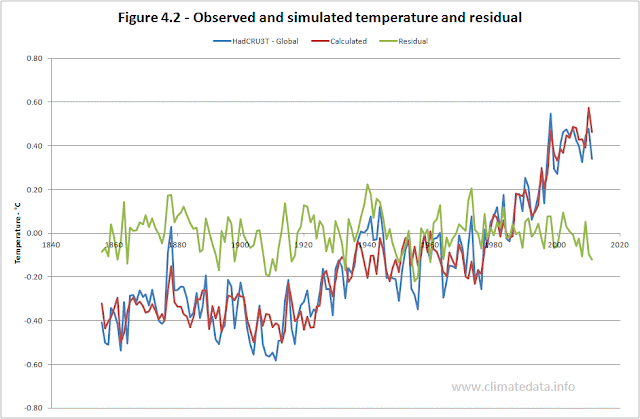In this case the residual has zero trend but the relatively high auto-correlation of 0.483. This suggests that model satisfactorily explains the trend but not all the variation in temperature.

The above is just a summary of a potentially much more detailed analysis: the conclusion is that the model is valid but that that its accuracy may be less than appears to be the case on a first glance at Figure 4.1.

Figure 4.3 shows the influence of each of the individual model parameters on global temperature.The two most important parameters in the model are GHG forcing, which explains the trend, and the AMO, which explains why the rate of temperature change appears to accelerate, as it did from 1910 to 1945 and from 1975 to 2005, and slow down, as it did from 1945 to 1975 and as it appears to be doing now. Over its cycle the AMO changes temperature by ± 0.20 °C. The extended TSI data I have used shows a slight upward trend, which is not as apparent in sunspot data, but the variation in temperature over a cycle is only around 0.05 °C. The effect of aerosols, and indirectly volcanoes, is minimal; less than 0.05 °C for Krakatau in 1882.

GHG forcing
The use of GHG forcing as the parameter which, effectively, explains the underlying trend is likely to be the most contentious (at least for some people). The value of the coefficient is 0.308 °C/watt/m2. This is equivalent to 3.25 W/m2/°C. This is close the accepted value of 3.2 W/m2/°C quoted by the IPCC (2008, Paragraph 8.6.2.2).

AMO
Several papers have described teleconnectioms between the AMO and geographical regions remote from its immediate locus. These cover large parts of the northern hemisphere and, in some papers, parts of the southern hemisphere. Whilst global temperatures and the AMO are mathematically linked (global temperatures include the effects of ocean temperatures and the AMO uses the difference between northern Atlantic and global ocean temperatures) published papers have considered it to be effectively independent of global temperature.

TSI
The coefficient for Total Solar Irradiance is 0.0365. Allowing for the fact that the sun only ‘sees’ a disk and the albedo of the earth is 0.7, this is equivalent to a global forcing of 0.208 °C/w/m2. This is similar to the figure for GHG forcing and for which similar comments therefore apply. The 95% errors bands for this coefficient are so high, ±0.046, however that the true figure might be closer to the theoretical value.

Aerosols
This is an area where there is no ‘right answer’, the IPCC reporting a wide range of values. The effect is due to volcanoes which have both a cooling effect (blocking out the sun) and a warming effect (heat absorption by black soot particles). The value of the effects it simulates is on the low side but within the range of IPCC models.

Components of Warming
The regression model allows the influence of each parameter to be calculated. The values for three periods are shown on figure 4.2.

Table 4.2 – Components of climate change

These figures suggest:

For the full period of calculation, 1856 to 2011, virtually the whole increase has been caused by the increase in GHGs.
For the last century, 1911 to 2011, 20% of the increase is due to the AMO and the remainder to GHGf.
For the period of rapid temperature rise from 1976 to 2005, half the increase was related to the AMO.
Aerosols and total solar radiation had very little impact on long-term temperature changes.
The warming from 1856 to 2011 due to GHG forcing is 0.777 °C. During that period CO2e increased from 289.6 ppm to 464.1 ppm. This represents a sensitivity to CO2e doubling of 1.14 °C which is very close to the accepted figure of 1 °C – without water vapour feedback. The figure for GHGf sensitivity of 3.25 W/m2/°C was also without water vapour feedback. If all the temperature increase from 1856 to the present gives an overall sensitivity to CO2e doubling of 1.12 °C this implies either that the sensitivity without water vapour feedback is lower than generally accepted and part of the increase is due to water vapour feedback or that there has been no water vapour feedback in the last 156 years. The latter would be surprising as there have been three periods of rapid temperature increase since 1850:

· 1858 to 1888, 0.54 °C, 0.18 °C/decade.
· 1911 to 1944, 0.70 °C, 0.21 °C/decade
· 1976 to 2005, 0.74 °C, 0.25 °C/decade.

I realise that the figure of 1°C for a CO2e doubling is less than the widely quoted figure of 3°C and I look at some of the implications of this in section 5.

Alternative values of the AMO

The above uses the estimate of the AMO from NOAA’s Earth System Research Laboratory, Physical Sciences Division. It was chosen as it the longest of those available. There is an alternative from the University Corporation for Atmospheric Research, National Center for Atmospheric Research which runs from 1870 to 2010. The two alternatives are shown in figure 4.4.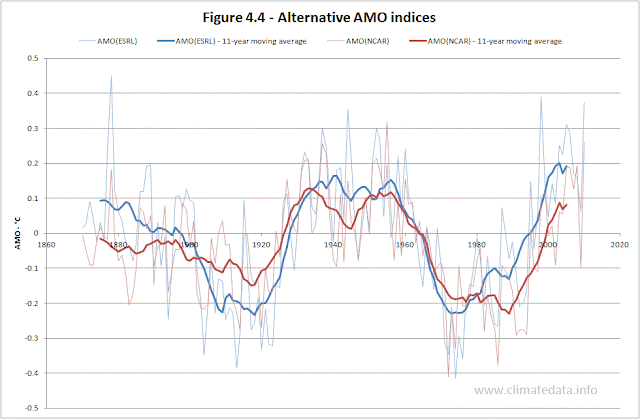As can be seen they are quite similar, particularly for later years when the data are more accurate. The difference in temperature calculation using the different indices is shown on Figure 4.5.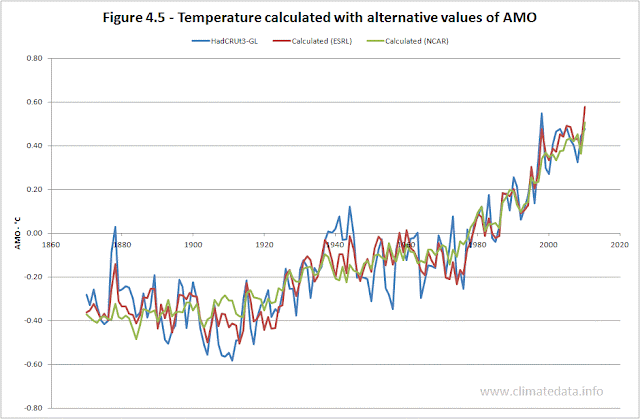Both sets of calculated flows are similar for much of the period. The main difference is in the period before 1950; with the NCAR value of the AMO the minimum around 1910 and the maximum around 1945 are not as well represented. This is to be expected from the form of the two AMO indices. Both models represent the slowdown in temperature increase at the start of this century. Table 4.3 shows coefficients for GHGf and TSI are similar those for the AMO and aerosols are different. The explained variance and the R2 are lower with the alternative AMO index.

Table 4.3 – Coefficients with alternative AMO indices (1870 to 2011)

5. Projections and comparison of regression model with IPCC models
Comparison with IPCC models

This chart (Figure 5.1) shows simulations with the regression model and a 23-model ensemble (downloaded from http://climexp.knmi.nl).Whilst neither model fully represents the low of 1911 nor the high of 1944 the regression model comes closest. Both models tend to increase for the period 1944 to 1960 but whereas the IPCC models have a rapid fall, due to the Agung volcano, and then rise, the regression model represents the local minimum in 1976 well. Both models then have similar accuracy until the start of the present century when the IPCC models show a continuing increase but the regression model simulates the slowdown in the temperature increase.

Temperatures without GHG forcing
One of the arguments in support of the models presented in the IPCC reports comes from a comparison of what the models simulated with GHG forcing and what they simulate without this.
Figure 5.2 shows the estimate presented by the IPCC and the estimate based on the regression model. The IPCC estimate was digitised from figure 9.5 of the IPCC report of 2005. The observed data were adjusted to have zero for the observed temperatures for the period 1950 to 2000.The differences between the two sets of calculated temperatures are large. In the case of the regression model the temperature without external forcing would follow the AMO derived trend with a mean of zero but some small effect from sun spots and volcanoes. In the case of the IPCC models the major positive forcing is from GHGs and the major negative forcing is from volcanoes. The green line shows four major falls in temperature, represented also to some extent in the regression model, and a steady increase from 1900 to 1960. The big difference is from the mid 1970s onwards. The regression model ‘shares’ the temperature increase between the AMO and GHGf; the IPCC models not only assumes the increase is driven solely by GHGf but at rate which also cancels out the large negative effect of volcanoes.

Projections
Much of the debate around climate change to date is about an appropriate response to the high temperature increases projected by IPCC models. If the projections of a temperature increase of 2 or 3 °C by the end of the century are correct then the costs of mitigation and/or adaptation might be justified; if the projections are overestimates then the costs are not justifiable.

To make a prediction it is necessary to be able to predict the forcing agents. I made three predictions and the way I predicted the forcing agents is given below.

1. Calibration from 1856 to 1933 (half the data period) and prediction from 1934 to 2011. The AMO used a sine curve fitted to the full period of data. The TSI used a typical sine curve but because of the irregular periodicity it was not fitted to historic data. Perfect foreknowledge of GHG forcing and aerosols was assumed. The synthetic curves are shown in Figures 5.3, for the AMO, Figure 5.4 for the TSI.

2. As above but calibration from 1856 to 1980 and prediction from 1981.

3. Prediction from 2011 to 2040. Assuming the same synthetic AMO and TSI values. Aerosols assumed to be zero. GHG forcing assumed to increase at same rate from 2012 onward as it did from 1981 to 2011.

Figure 5.3 shows the assumed curve for the AMO for prediction. It was derived by fitting a sine curve to the AMO data. I am not, of course, assuming that the AMO is that regular but as an estimate for a prediction it is as likely as any other. The shape of the curve suggests that the AMO might be at a peak and that it will decline over the coming decades; certainly AMO index has levelled off in recent years.For the TSI it was not possible to fit a sine curve as its periodicity is not constant. I therefore developed a synthetic record which retains many of the characteristics of observed TSI variations which is shown on figure 5.4.The first prediction was made in 1934, using half the data to calibrate the model and half for the prediction. For this period the R2 value was 0.52, much less than for the whole period. The prediction gets the general shape correct but underestimates the 2011 temperature. This was due to the fact that the coefficient for GHGf was 0.242 °C/watt/m2rather than 0.308 °C/watt/m2 when calibrated on the whole period. Given that only 20% of the greenhouse gas forcing for the whole period occurred before 1934 it is understandable that this parameter is not as accurate.

The second prediction was made in 1980, calibrated on data up to that year. This prediction accurately predicted the increase in temperature over that period and the recent levelling off.

The third prediction used the full period for calibration, with GHG forcing estimated to increase at the same rate for the next 30 years as it had over the previous 30 years. On the basis of the assumptions set out above, the regression model projects a temperature increase from 2012 to 2040 of 0.098 °C.

By way of comparison I have added a projection based on the 23-model IPCC ensemble using the a1b, 'business as usual', scenario. For the same period it projects in increase of 0.77 °C.The difference in the projections for the future represents the largest difference between the regression model and IPCC models. Which model proves correct only time will tell?

6. AMO and other climate parameters
Sea Level
Figure 6.1 shows the AMO and global sea level. The sea levels are a composite of tide gauge and satellite data. At a first glance there appears to be little correspondence between the two.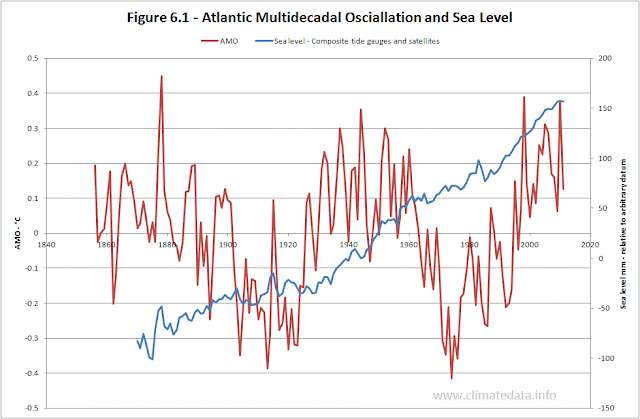Figure 6.2 presents the same data expressed as a 20-year trend for the sea levels and an 11 year moving average for the AMO. The trends were calculated using the LINEST function in Excel and represent the rate of increase for each 20-year period preceding the date on the chart.What this chart suggests is that the rate of sea level rise is influenced by the AMO but with a lag of a decade or so. It shows that the rate of sea level rise reached a minimum close to 0 mm/year in the 1930s and a minimum of around 1 mm/year in the 1980s. It is possible that under the influence of the AMO the rate of rise will fall to 2 to 2.5 mm/year in the coming decades.

There is a corollary to the above. One of the key points in the debate about climate is the thermal inertia in the system. It is argued that while the atmosphere responds fairly rapidly to increases in GHGs it take longer for the sea to respond and this delays the impact of the GHGs. Since sea levels seem to respond with a lag of a decade or two to the AMO, and sea levels are in part a function of ocean temperature, this might suggest that the delaying effect of the oceans is of the same order of magnitude.

Hurricanes
Figure 6.3 shows the AMO and the Accumulated Cyclone Energy (ACE) for Atlantic tropical storms.Given that storms rise in part of the area over which the AMO is calculated the correspondence is not high in particular there is a period around 1940 when the AMO was at its peak but storm energy was low. A trend line through the annual series of ACE suggest an increase of 3.6 10-4 km2 per decade. Whilst it is possible that a declining AMO will lead a reduction in the energy of tropical storms for the next few decades the overall trend seems to be for a steady increase.

7. Conclusions

The American journalist H. L. Mencken once said “There is always a well-known solution to every human problem--neat, plausible, and wrong.” What I have presented above is based on a well-known method, multiple-linear regression. To represent the variation in global temperatures with a few parameters more accurately than the powerful AOGCMs is pretty neat. That the parameters of the model are close to what might be expected makes it plausible. But, is it wrong?

There is no doubt about parts of the model. The regression analysis was done using Excel (LINEST and the REGRESSION component of the Data Analysis tools of Excel) with alternative temperature and forcing data sets with very similar results. So the statement that the model explains almost 90% of the variance in annual temperatures is certainly correct. So what could be wrong?

I have already considered the auto-correlation of the data. It is true that the data are highly auto-correlated. This applies not only to the HadCRUT3 temperature but also the independent variables – particularly the greenhouse gas forcing. A simple way of analysing the effective length of the data record (n * (1-r1)/(1+r1) where n is the number of data items and r1 is the serial correlation) suggests that the length of the data record is effectively only 7.8 years. However the R2and F values are so high that the probability of the result occurring by chance is still effectively zero.

Another possible factor is the fact that the one of the independent variables, the AMO, is indirectly related to the dependent variable, global temperature. The AMO is based on the difference between the temperature in the Atlantic between 0° and 70° N and the ocean temperature. The HadCRUT3 temperature record is based on land temperature and ocean temperatures. The correlation coefficient between the AMO and global temperature is 0.41, which means that there is a degree of interdependence. However the effect of this is that the accuracy is artificially enhanced rather than the model being invalidated. As a simple way of examining this, the model was run with the synthetic AMO replacing the observed AMO. The result is shown on figure 7.1In this case the explained variance was 83.5%, a bit lower than when using the original AMO data. This shows that without some of the short term variation the regression model still tracks the temperature trend closely. Also on the same chart I show the results of the IPCC 23-model ensemble. For most of the time the two simulations are very similar. The period where they are different, roughly 1930 to 1960, is crucial to understanding why they give different projections. From 1910 to 1950 the AMO was increasing. This was picked up by the regression model and not the IPCC models. Similar considerations apply to the period from 1975 to 2005 but in a more subtle way. In the regression model half the increase in temperature is due to the increase in the AMO and half due to GHGf; with the IPCC ensemble all of the increase, in fact slightly more than all as there is an assumption that the natural trend was negative due to volcanoes, is due to GHGf.

THe coefficients for each of the variables were similar to the original values except the one for Aerosols whih increased to -0.78 (from -0.28. I have already that the AMO, since it represented the 1998 temperature peak carried an 'imprint' of the ENSO. It appears that it also carries an 'imprint' of the effect of volcanoes.

The main charge against the regression model however is likely to be the fact that it does not take account of water vapour feedback. The fact the coefficient for GHGf and sensitivity to CO2e doubling are both close to the accepted no-feedback values would not be considered a defence. There are two possible responses. The first is that sea levels, in part a function of ocean temperature, respond a decade or so after changes to the AMO. The second is that temperatures were already levelling off as the AMO approached its maximum. There is also the fact, mentioned earlier; that either there has been no water vapour feedback over the last century and a half or part of the increase is due to water vapour feedback, in which case the sensitivity to CO2e doubling is less than assumed.

A further objection is that the model assume an instaneous response and ignores thermal inertia (effectively that of the oceans). I have already commented on the fact that the rate of change of sea level seems to respond to the AMO. Sea surface temperatures have been effectively flat for from 2000 to 2011 - again suggesting that they may be levelling of in response to the AMO.

Another possible objection is that model is over simplistic ignoring many of the complex interchanges and feedbacks which take place. For example the transfer of CO2 between the atmosphere and the ocean is complex. As ocean temperature rises the ability of the oceans to absorb CO2 decreases which could provide a positive feedback mechanism. However other factors also change with increased ocean temperature such as the dynamics of mixing and this in turn can affect phytoplankton and CO2 uptake from photosynthesis.

I have suggested that if the model is correct it is likely that temperatures will rise by only a fraction of the amount predicted by the IPCC model ensemble. That prediction depends on the assumptions used in the projection. The effect of TSI (sunspots) and volcanoes is quite small and are unlikely to add to the significantly to the increase. If there is a major volcano, or volcanoes, then the rise will be smaller. It is also widely accepted the amplitude of sunspot cycles will be diminishing in coming decades. The two critical assumptions are those related the future of GHGf and the evolution of the AMO.

The projection of GHGf forcing included in the 2005 Assessment Report was higher than has been the case. It also predicted that the effect of the GHG would increase to about 2040 and then decline, in part to due to weakening effect of the CFCs which are being phased out under the Montreal Protocol. At present most of the increase in CO2 emission is being driven by coal fired power stations in countries such as China and India whilst in the US, thanks to the lower emission per unit energy of shale gas, CO2 emissions are declining. Although European policies at the moment tend to favour renewable sources (principally wind) it is likely that the shale gas deposits in Europe, present in at least 12 countries, will be exploited in the next decade or so, if only to decrease reliance on imports from Russia and the Middle-East. China and India also have large potential reserves of shale gas. In other words the assumption that CO2e would increase at the same rate in the future as over the previous 30 years is probably reasonable.

The big unknown is the future evolution of the AMO. The sine curve assumption gives quite a good fit (R²=0.47) but it would be unrealistic to assume that the future will necessarily reflect the past. Grey at al (2004) developed AMO estimates based on tree rings from 1567 to 1990. These suggest that when the AMO reaches the level it is at now and is starting to level off then it continues to decline over coming decades. It is of course possible to track the AMO and revise the projections accordingly.

Those of you who have read this far may have one last question. What will happen after 2040? If, even approximately, the AMO follows past patterns it will be starting to increase. The model suggests that temperature will start to increase at rate similar to those of the 1980s and 1990s. The total increase would still be less that IPCC models currently project. However if the regression model is correct humanity would have 30 years with little increase in temperature to prepare for it.